StatLect

# Marginal distribution function

Given a random vector, the probability distribution of all its components, considered together, is called joint distribution, while the probability distribution of one of its components, considered in isolation, is called marginal distribution.## Definition

The following is a more precise definition.

Definition Let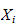be the-th component of a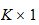random vector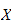having joint distribution function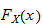. The distribution function ofis called marginal distribution function ofand it is denoted by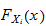.

## Marginal distributions and independence

Marginal distribution functions play an important role in the characterization of independence between random variables: two random variables are independent if and only if their joint distribution function is equal to the product of their marginal distribution functions (see the lecture entitled Independent random variables).

Example Letandbe two random variables having marginal distribution functions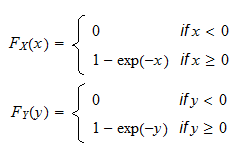and joint distribution function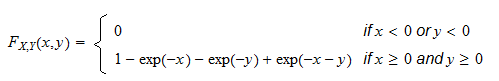It is easy to check that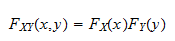for any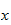and, which implies thatandare independent.

## More details

A more detailed discussion of the marginal distribution function can be found in the lecture entitled Random vectors.

## Keep reading the glossary

Previous entry: Loss function

Next entry: Marginal probability density function